Latest Banking jobs   »   Quantitative Aptitude Quiz For SIDBI Grade...

# Quantitative Aptitude Quiz For SIDBI Grade A Prelims 2023- 16th January

Directions (1-15):In each of these questions, two equation (I) and (II) are given. You have to solve both the equations and give answer.

Q1. I. x² + 19x – 92 = 0
II. y² + 46y + 529 = 0
(a) If x > y
(b) If x ≥ y
(c) If x < y
(d) If x ≤ y
(e) If x = y or no relation can be established between x and y

Q2. I. 2x² – 3x – 9 = 0
II. y² – 29y + 54 = 0
(a) If x > y
(b) If x ≥ y
(c) If x < y
(d) If x ≤ y
(e) If x = y or no relation can be established between x and y

Q3. I. 3x² – 6x + 12 = 7x
II. 4y² – 1y – 12 = 1y
(a) If x > y
(b) If x ≥ y
(c) If x < y
(d) If x ≤ y
(e) If x = y or no relation can be established between x and y

Q4. I. 6x² – 5x – 4 = 0
II. 4y² – 16y + 15 = 0
(a) If x > y
(b) If x ≥ y
(c) If x < y
(d) If x ≤ y
(e) If x = y or no relation can be established between x and y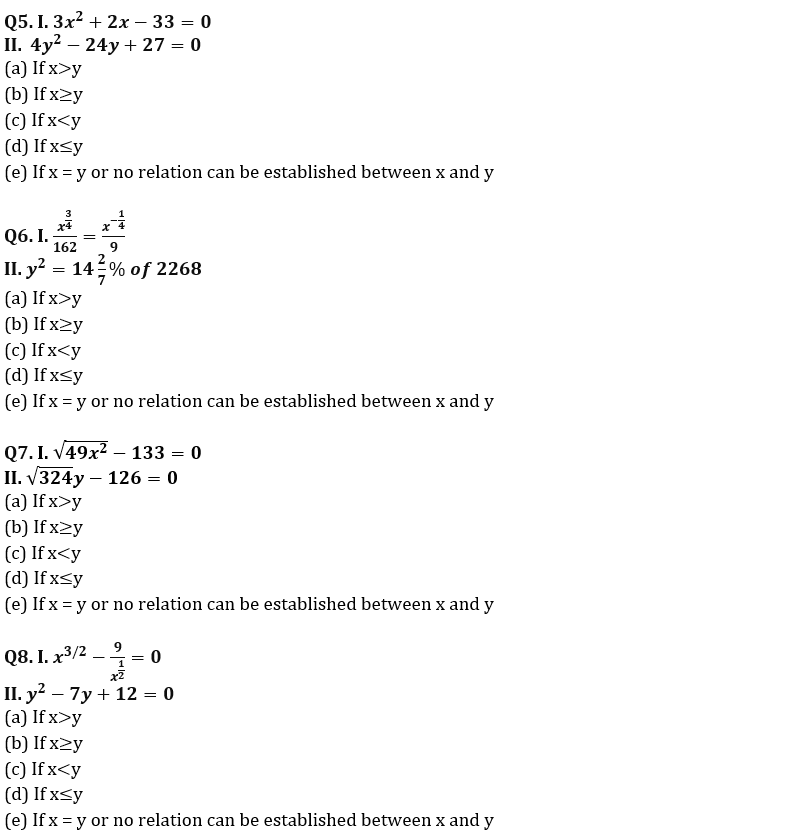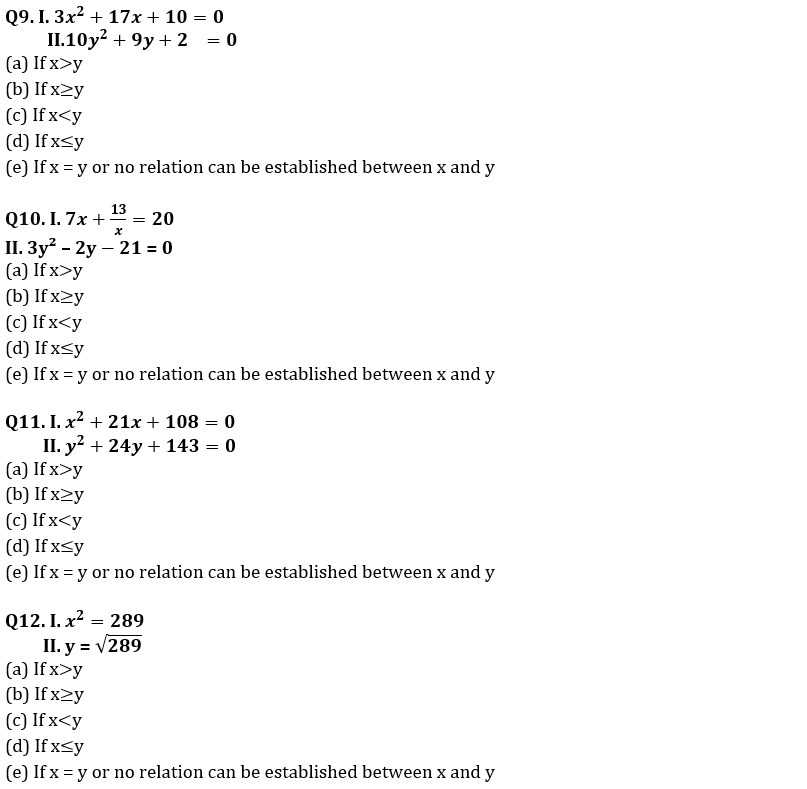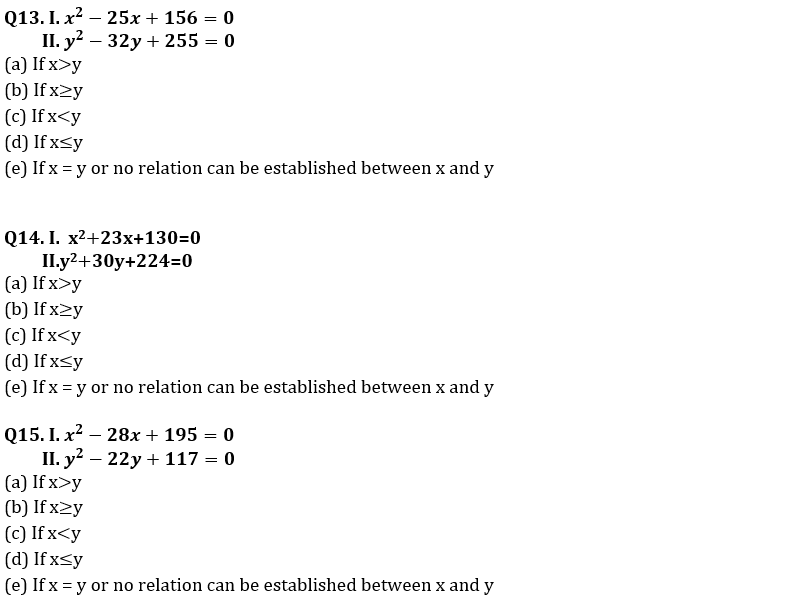Solutions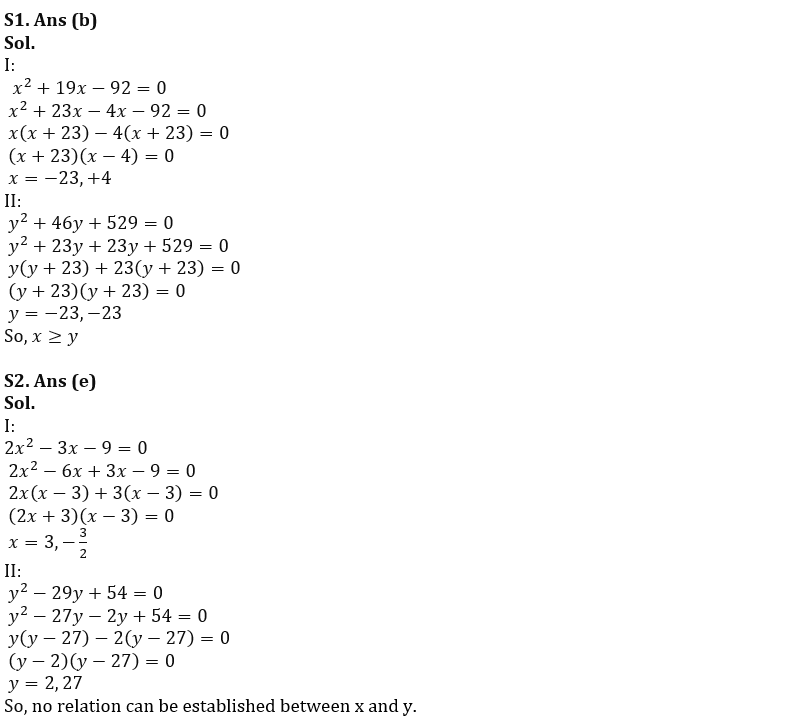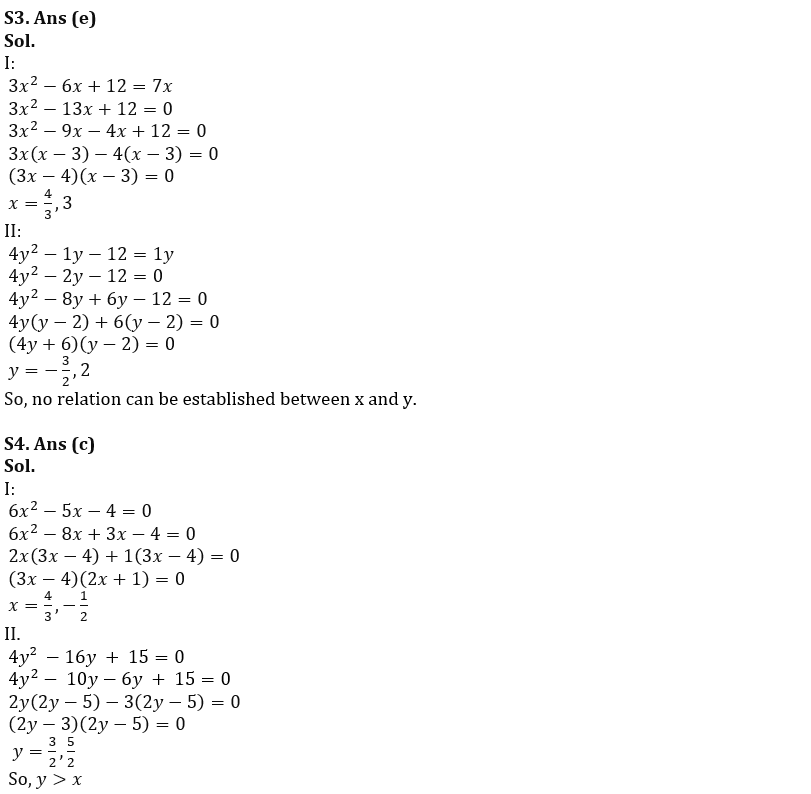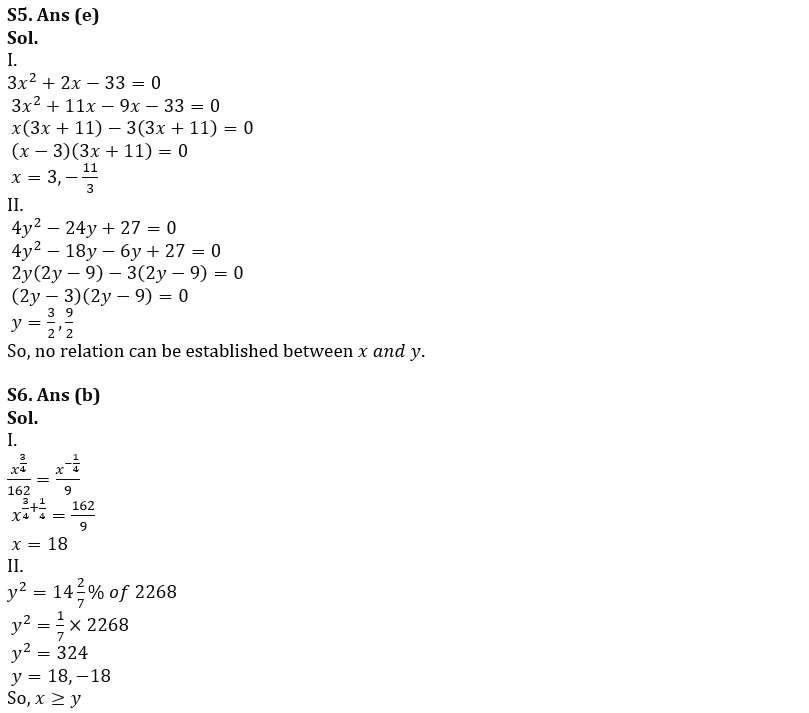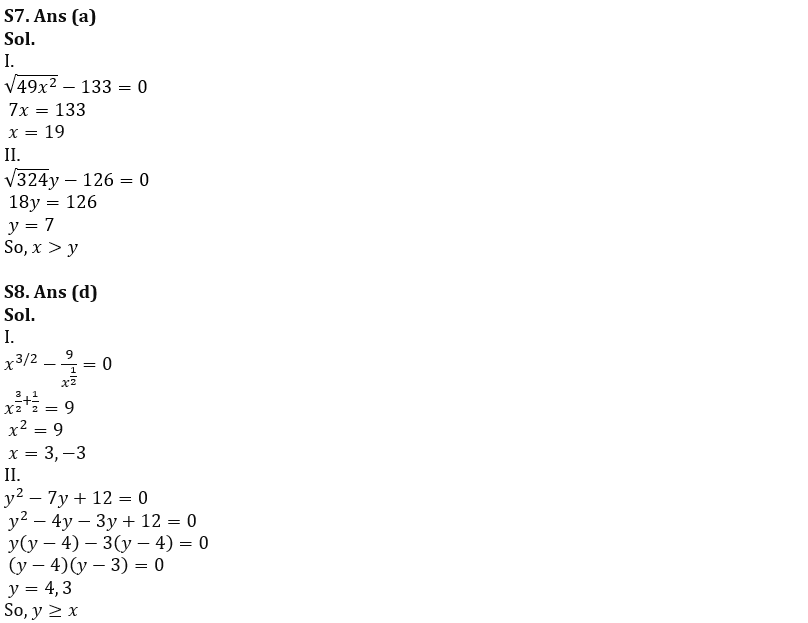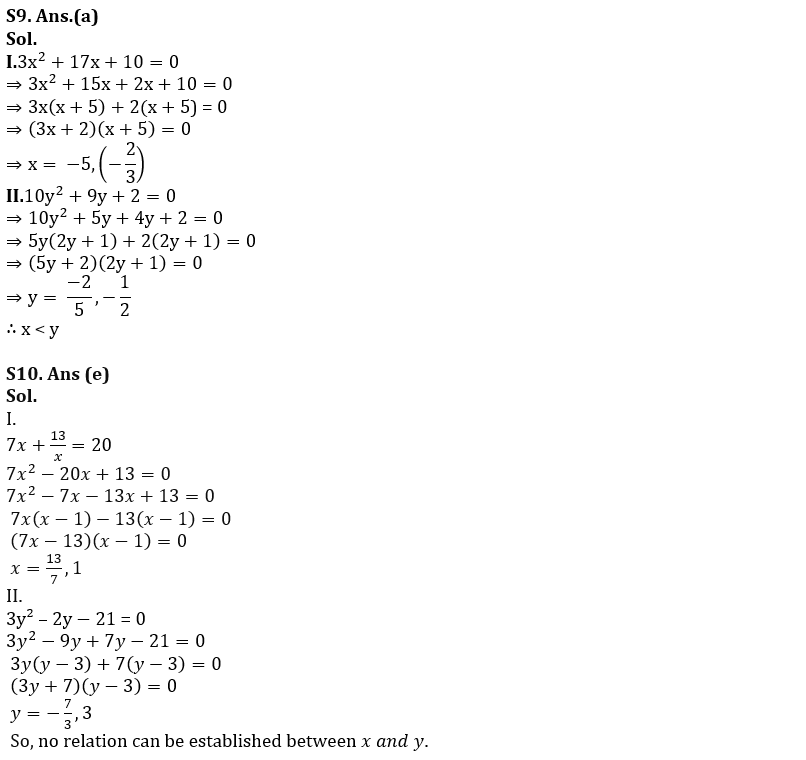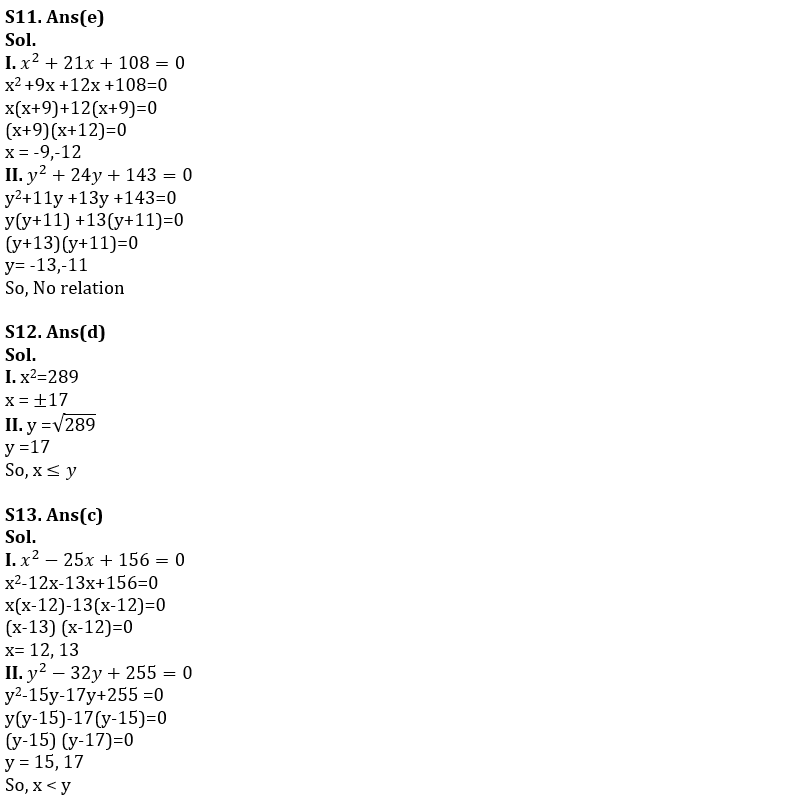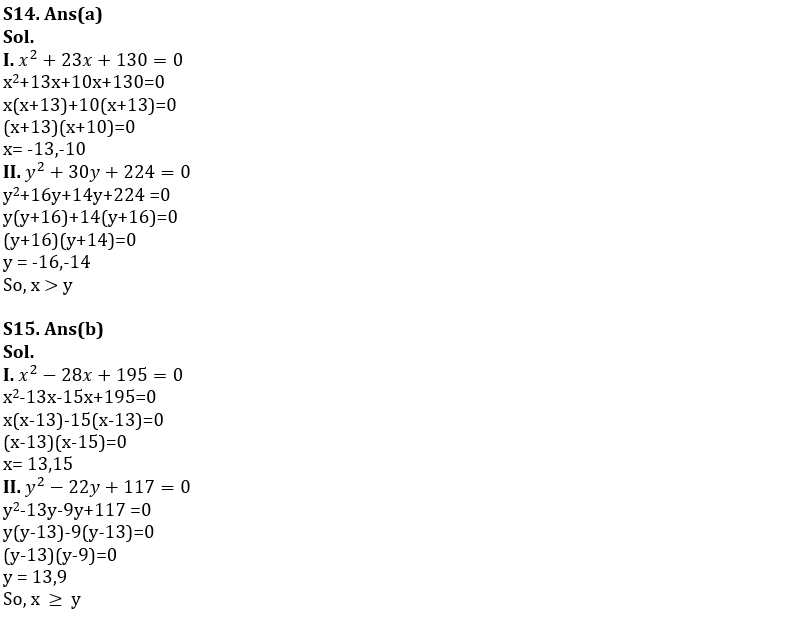## FAQs

### When will the SIDBI Grade A Exam 2023 be held?

The SIDBI Grade A Exam 2023 will be held on 28th January 2023.

#### Congratulations!Union Budget 2023-24: Free PDF